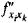# Relative Extremum

The following article is from The Great Soviet Encyclopedia (1979). It might be outdated or ideologically biased.

## Relative Extremum

a maximum or minimum value of a continuous function. More precisely, a function f(x) that is continuous at a point x0 has a relative maximum at x0 if in the domain of f(x) there exists a neighborhood (x0 + δ, x0 – δ) of x0 such that the inequality f(x0) ≥ f(x) is fulfilled at all points of the neighborhood. Similarly, f(x) has a relative minimum at x0 if there is a neighborhood such that the inequality f(x0) ≤ f(x) holds for all its points.

A relative maximum at x0 is said to be proper if there exists a neighborhood in which f(x0) > f(x) when xx0; the term “proper” is applied to a relative minimum at x0 if there is a neighborhood in which f(x0) < f(x) when xx0. The function shown in Figure 1 has a proper maximum at point A; although the function’s value at point B is a minimum, it is not a proper minimum.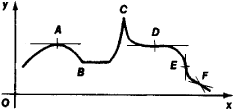Figure 1

A point at which a function has a relative maximum is called a maximum point, and a point at which it has a relative minimum is called a minimum point. In order for a function f(x) to have a relative extremum at some point x0, it must be continuous at x0; in addition, the derivative f(x0) must either be equal to zero, as at point A in Figure 1, or be nonexistent, as at point C. The following statements present sufficient conditions for relative extrema: (1) if in some neighborhood of x0 the derivative is positive to the left of x0 and negative to the right of x0, then f(x) has a relative maximum at x0; (2) if f′(x) is negative to the left of x0 and positive to the right, then f(x0) is a relative minimum. If, however, f′(x) does not change sign in the neighborhood of x0, then f(x) does not have an extremum at x0; examples of such points are D, E, and Fin Figure 1.

Suppose f(x) has n successive derivatives at x0. If f′(x0) = f′(x0) = . . . = f(n – 1)(x0) = 0 and f(n)(x0) ≠ 0, then f(x) does not have a relative extremum at x0 if n is odd and does have a relative extremum if n is even. The extremum is a minimum if f(n)(x0) > 0 and a maximum if f(n)(x0) < 0.

If f(x) has a relative maximum at x0 and if f(x0) ≥ f(x) for all xx0 in the domain of the function, then f(x0) is called an absolute maximum. If f(x) has a relative minimum at x0 and if f(x0) ≤ f(x) for all xx0, then f(x0) is said to be an absolute minimum.

A relative extremum of a function of several variables is defined in much the same way as a relative extremum of a function of a single variable. A necessary condition for a function of several variables to have a relative extremum is that the first-order partial derivatives be equal to zero or be nonexistent. For example, in Figure 2,a they are equal to zero at point M, and in Figure 2,b they do not exist at M.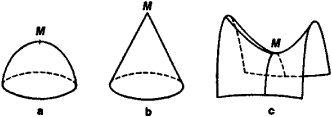Figure 2

Suppose that in some neighborhood of the point M(x0, y0) there exist continuous first-and second-order partial derivatives of the function f(x, y). If at M we have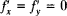and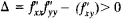, then f(x, y) has a relative extremum at M. The extremum is a maximum if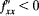and a minimum if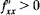. A relative extremum does not exist at M if Δ < 0; in this case M is called a saddle point (see Figure 2,c).

The sufficient conditions for a relative extremum of a function of many variables reduce to the quadratic form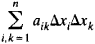being positive or negative definite; here, aik is the value of